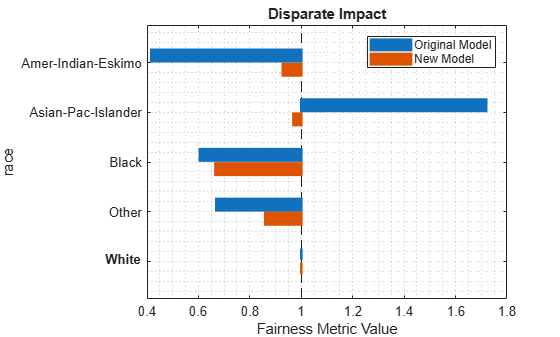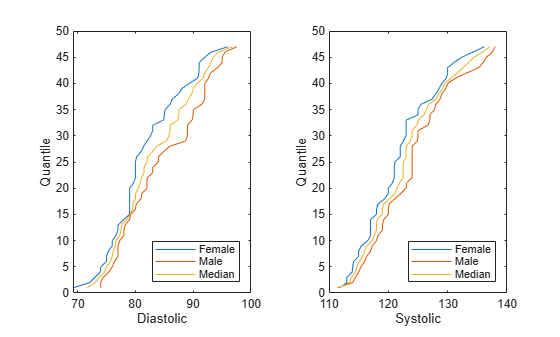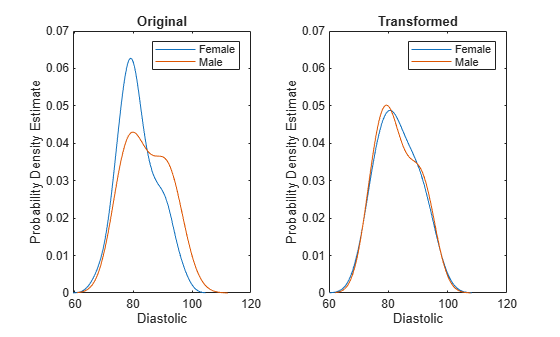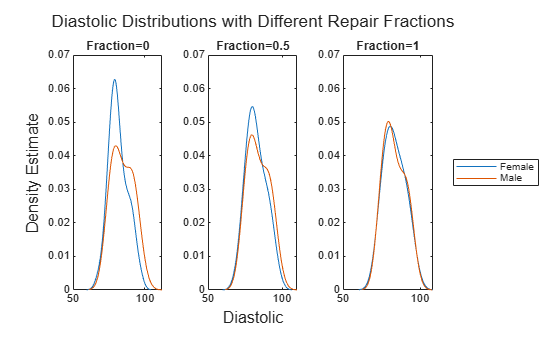# disparateImpactRemover

Remove disparate impact of sensitive attribute

Since R2022b

## Description

To try to create fairness in binary classification, you can use the `disparateImpactRemover` function to remove or reduce the disparate impact of a sensitive attribute. Before training your model, use the sensitive attribute to transform the continuous predictors in the training data set. The function returns the transformed data set and a `disparateImpactRemover` object that contains the transformation. Pass the transformed data set to an appropriate training function, such as `fitcsvm`, and pass the object to the `transform` object function to apply the transformation to a new data set, such as a test data set.

Note

You must transform new data, such as test data, after training a model using `disparateImpactRemover`. Otherwise, the predicted results are inaccurate.

## Creation

### Syntax

``remover = disparateImpactRemover(Tbl,AttributeName)``
``[remover,transformedData] = disparateImpactRemover(Tbl,AttributeName)``
``[remover,transformedData] = disparateImpactRemover(X,attribute)``
``[remover,transformedData] = disparateImpactRemover(___,Name=Value)``

### Description

````remover = disparateImpactRemover(Tbl,AttributeName)` removes the disparate impact of the `AttributeName` sensitive attribute in the table `Tbl` by transforming the continuous predictors in the data set `Tbl`. The returned `disparateImpactRemover` object (`remover`) stores the transformation, which you can apply to new data. For more information, see Algorithms.```

example

````[remover,transformedData] = disparateImpactRemover(Tbl,AttributeName)` also returns the transformed predictor data `transformedData`, which corresponds to the data in `Tbl`.Note that `transformedData` includes the sensitive attribute in this syntax. After using `disparateImpactRemover`, avoid using the sensitive attribute as a separate predictor when training your model.```

example

````[remover,transformedData] = disparateImpactRemover(X,attribute)` uses the numeric predictor data `X` and the sensitive attribute specified by `attribute` to transform the predictors.```

example

````[remover,transformedData] = disparateImpactRemover(___,Name=Value)` specifies options using one or more name-value arguments in addition to any of the input argument combinations in previous syntaxes. For example, you can specify the extent of the data transformation by using the `RepairFraction` name-value argument. A value of 1 indicates a full transformation, and a value of 0 indicates no transformation.```

### Input Arguments

expand all

Data set, specified as a table. Each row of `Tbl` corresponds to one observation, and each column corresponds to one variable. When you use a table with `disparateImpactRemover`, the table must include the sensitive attribute. The table can include additional variables, such as the response variable. Multicolumn variables and cell arrays other than cell arrays of character vectors are not allowed.

If `Tbl` contains numeric variables that you want `disparateImpactRemover` to ignore (such as observation weights), you can specify the continuous numeric variables to transform by using the `PredictorNames` name-value argument.

Data Types: `table`

Sensitive attribute name, specified as the name of a variable in `Tbl`. You must specify `AttributeName` as a character vector or a string scalar. For example, if the sensitive attribute is stored as `Tbl.Attribute`, then specify it as `"Attribute"`.

The sensitive attribute must be a numeric vector, logical vector, character array, string array, cell array of character vectors, or categorical vector.

Data Types: `char` | `string`

Predictor data, specified as a numeric matrix. Each row of `X` corresponds to one observation, and each column corresponds to one predictor variable. `X` and `attribute` must have the same number of rows.

To specify the names of the predictors in the order of their appearance in `X`, use the `PredictorNames` name-value argument.

Data Types: `single` | `double`

Sensitive attribute, specified as a numeric column vector, logical column vector, character array, string array, cell array of character vectors, or categorical column vector.

• If `attribute` is an array, then each row of the array must correspond to a group in the sensitive attribute.

• `attribute` and `X` must have the same number of rows.

Data Types: `single` | `double` | `logical` | `char` | `string` | `cell` | `categorical`

Name-Value Arguments

Specify optional pairs of arguments as `Name1=Value1,...,NameN=ValueN`, where `Name` is the argument name and `Value` is the corresponding value. Name-value arguments must appear after other arguments, but the order of the pairs does not matter.

Example: `disparateImpactRemover(Tbl,"Age",PredictorNames=["Diastolic","Systolic"])` specifies to transform the `Diastolic` and `Systolic` variables in the table `Tbl` by using the `Age` sensitive attribute in `Tbl`.

Names of the predictor variables to transform, specified as a string array of unique names or cell array of unique character vectors.

• If you supply `Tbl`, then you can use `PredictorNames` to specify which numeric predictor variables to transform.

• If you supply `X`, then you can use `PredictorNames` to assign names to the predictor variables in `X`.

Example: `PredictorNames=["SepalLength","SepalWidth","PetalLength","PetalWidth"]`

Data Types: `string` | `cell`

Fraction of the data transformation, specified as a numeric scalar in the range [0,1]. A value of 1 indicates a full transformation, and a value of 0 indicates no transformation.

A greater repair fraction can result in a greater loss in model prediction accuracy. For more information, see .

Example: `RepairFraction=0.5`

Data Types: `single` | `double`

### Output Arguments

expand all

Predictor data transformer, returned as a `disparateImpactRemover` object. `remover` contains the transformation of the `remover.PredictorNames` predictor variables with respect to the `remover.SensitiveAttribute` variable.

Transformed predictor data corresponding to the data in `Tbl` or `X`, returned as a table or numeric matrix. Note that `transformedData` can include the sensitive attribute. After you use the `disparateImpactRemover` function, avoid using the sensitive attribute as a separate predictor when training your model.

## Properties

expand all

Fraction of the data transformation, returned as a numeric scalar in the range [0,1]. A value of 1 indicates a full transformation, and a value of 0 indicates no transformation.

If you want to adjust the repair fraction after creating a `disparateImpactRemover` object, specify the `RepairFraction` name-value argument of the `transform` object function.

Data Types: `single` | `double`

Names of the transformed predictor variables, returned as a cell array of unique character vectors. The order of the elements of `PredictorNames` corresponds to the order in which the predictor names appear in the `Tbl` or `X` data.

Data Types: `cell`

Sensitive attribute, returned as a variable name, numeric column vector, logical column vector, character array, cell array of character vectors, or categorical column vector.

• If you use a table to create the `disparateImpactRemover` object, then `SensitiveAttribute` is the name of the sensitive attribute. The name is stored as a character vector.

• If you use a matrix to create the `disparateImpactRemover` object, then `SensitiveAttribute` has the same size and data type as the sensitive attribute used to create the object. (The software treats string arrays as cell arrays of character vectors.)

Data Types: `single` | `double` | `logical` | `char` | `cell` | `categorical`

## Object Functions

 `transform` Transform new predictor data to remove disparate impact

## Examples

collapse all

Train a binary classifier, classify test data using the model, and compute the disparate impact for each group in the sensitive attribute. To reduce the disparate impact values, use `disparateImpactRemover`, and then retrain the binary classifier. Transform the test data set, reclassify the observations, and compute the disparate impact values.

Load the sample data `census1994`, which contains the training data `adultdata` and the test data `adulttest`. The data sets consist of demographic information from the US Census Bureau that can be used to predict whether an individual makes over \$50,000 per year. Preview the first few rows of the training data set.

```load census1994 head(adultdata)```
``` age workClass fnlwgt education education_num marital_status occupation relationship race sex capital_gain capital_loss hours_per_week native_country salary ___ ________________ __________ _________ _____________ _____________________ _________________ _____________ _____ ______ ____________ ____________ ______________ ______________ ______ 39 State-gov 77516 Bachelors 13 Never-married Adm-clerical Not-in-family White Male 2174 0 40 United-States <=50K 50 Self-emp-not-inc 83311 Bachelors 13 Married-civ-spouse Exec-managerial Husband White Male 0 0 13 United-States <=50K 38 Private 2.1565e+05 HS-grad 9 Divorced Handlers-cleaners Not-in-family White Male 0 0 40 United-States <=50K 53 Private 2.3472e+05 11th 7 Married-civ-spouse Handlers-cleaners Husband Black Male 0 0 40 United-States <=50K 28 Private 3.3841e+05 Bachelors 13 Married-civ-spouse Prof-specialty Wife Black Female 0 0 40 Cuba <=50K 37 Private 2.8458e+05 Masters 14 Married-civ-spouse Exec-managerial Wife White Female 0 0 40 United-States <=50K 49 Private 1.6019e+05 9th 5 Married-spouse-absent Other-service Not-in-family Black Female 0 0 16 Jamaica <=50K 52 Self-emp-not-inc 2.0964e+05 HS-grad 9 Married-civ-spouse Exec-managerial Husband White Male 0 0 45 United-States >50K ```

Each row contains the demographic information for one adult. The last column `salary` shows whether a person has a salary less than or equal to \$50,000 per year or greater than \$50,000 per year.

Remove observations from `adultdata` and `adulttest` that contain missing values.

```adultdata = rmmissing(adultdata); adulttest = rmmissing(adulttest);```

Specify the continuous numeric predictors to use for model training.

```predictors = ["age","education_num","capital_gain","capital_loss", ... "hours_per_week"];```

Train an ensemble classifier using the training set `adultdata`. Specify `salary` as the response variable and `fnlwgt` as the observation weights. Because the training set is imbalanced, use the `RUSBoost` algorithm. After training the model, predict the salary (class label) of the observations in the test set `adulttest`.

```rng("default") % For reproducibility mdl = fitcensemble(adultdata,"salary",Weights="fnlwgt", ... PredictorNames=predictors,Method="RUSBoost"); labels = predict(mdl,adulttest);```

Transform the training set predictors by using the `race` sensitive attribute.

```[remover,newadultdata] = disparateImpactRemover(adultdata, ... "race",PredictorNames=predictors); remover```
```remover = disparateImpactRemover with properties: RepairFraction: 1 PredictorNames: {'age' 'education_num' 'capital_gain' 'capital_loss' 'hours_per_week'} SensitiveAttribute: 'race' ```

`remover` is a `disparateImpactRemover` object, which contains the transformation of the `remover.PredictorNames` predictors with respect to the `remover.SensitiveAttribute` variable.

Apply the same transformation stored in `remover` to the test set predictors. Note: You must transform both the training and test data sets before passing them to a classifier.

```newadulttest = transform(remover,adulttest, ... PredictorNames=predictors);```

Train the same type of ensemble classifier as `mdl`, but use the transformed predictor data. As before, predict the salary (class label) of the observations in the test set `adulttest`.

```rng("default") % For reproducibility newMdl = fitcensemble(newadultdata,"salary",Weights="fnlwgt", ... PredictorNames=predictors,Method="RUSBoost"); newLabels = predict(newMdl,newadulttest);```

Compare the disparate impact values for the predictions made by the original model (`mdl`) and the predictions made by the model trained with the transformed data (`newMdl`). For each group in the sensitive attribute, the disparate impact value is the proportion of predictions in that group with a positive class value (${p}_{g+}$) divided by the proportion of predictions in the reference group with a positive class value (${p}_{r+}$). An ideal classifier makes predictions where, for each group, ${p}_{g+}$ is close to ${p}_{r+}$ (that is, where the disparate impact value is close to 1).

Compute the disparate impact values for the `mdl` predictions and the `newMdl` predictions by using `fairnessMetrics`. Include the observation weights. You can use the `report` object function to display bias metrics, such as disparate impact, that are stored in the `evaluator` object.

```evaluator = fairnessMetrics(adulttest,"salary", ... SensitiveAttributeNames="race",Predictions=[labels,newLabels], ... Weights="fnlwgt",ModelNames=["Original Model","New Model"]); evaluator.PositiveClass```
```ans = categorical >50K ```
`evaluator.ReferenceGroup`
```ans = 'White' ```
`report(evaluator,BiasMetrics="DisparateImpact")`
```ans=5×5 table Metrics SensitiveAttributeNames Groups Original Model New Model _______________ _______________________ __________________ ______________ _________ DisparateImpact race Amer-Indian-Eskimo 0.41702 0.92804 DisparateImpact race Asian-Pac-Islander 1.719 0.9697 DisparateImpact race Black 0.60571 0.66629 DisparateImpact race Other 0.66958 0.86039 DisparateImpact race White 1 1 ```

For the `mdl` predictions, several of the disparate impact values are below the industry standard of 0.8, and one value is above 1.25. These values indicate bias in the predictions with respect to the positive class `>50K` and the sensitive attribute `race`.

The disparate impact values for the `newMdl` predictions are closer to 1 than the disparate impact values for the `mdl` predictions. One value is still below 0.8.

Visually compare the disparate impact values by using the bar graph returned by the `plot` object function.

`plot(evaluator,"DisparateImpact")`The `disparateImpactRemover` function seems to have improved the model predictions on the test set with respect to the disparate impact metric.

Check whether the transformed predictors negatively affect the accuracy of the model predictions. Compute the accuracy of the test set predictions for the two models `mdl` and `newMdl`.

`accuracy = 1-loss(mdl,adulttest,"salary")`
```accuracy = 0.8024 ```
`newAccuracy = 1-loss(newMdl,newadulttest,"salary")`
```newAccuracy = 0.7955 ```

The model trained using the transformed predictors (`newMdl`) achieves similar test set accuracy compared to the model trained with the original predictors (`mdl`).

Try to remove the disparate impact of a sensitive attribute by adjusting continuous numeric predictors. Visualize the difference between the original and adjusted predictor values.

Suppose you want to create a binary classifier that predicts whether a patient is a smoker based on the patient's diastolic and systolic blood pressure values. Also, you want to remove the disparate impact of the patient's gender on model predictions. Before training the model, you can use `disparateImpactRemover` to transform the continuous predictor variables in your data set.

Load the `patients` data set, which contains medical information for 100 patients. Convert the `Gender` and `Smoker` variables to `categorical` variables. Specify the descriptive category names `Smoker` and `Nonsmoker` rather than `1` and `0`.

```load patients Gender = categorical(Gender); Smoker = categorical(Smoker,logical([1 0]), ... ["Smoker","Nonsmoker"]);```

Create a matrix containing the continuous predictors `Diastolic` and `Systolic`.

`X = [Diastolic,Systolic];`

Find the observations in the two groups of the sensitive attribute `Gender`.

```femaleIdx = Gender=="Female"; maleIdx = Gender=="Male"; femaleX = X(femaleIdx,:); maleX = X(maleIdx,:);```

Compute the `Diastolic` and `Systolic` quantiles for the two groups in the sensitive attribute. Specify the number of quantiles to be the minimum number of group observations across the groups in the sensitive attribute, provided that the number is smaller than 100.

```t = tabulate(Gender); t = array2table(t,VariableNames=["Value","Count","Percent"])```
```t=2×3 table Value Count Percent __________ ______ _______ {'Female'} {} {} {'Male' } {} {} ```
`numQuantiles = min(100,min(t.Count{:}))`
```numQuantiles = 47 ```
```femaleQuantiles = quantile(femaleX,numQuantiles,1); maleQuantiles = quantile(maleX,numQuantiles,1);```

Compute the median quantiles across the two groups.

```Q(:,:,1) = femaleQuantiles; Q(:,:,2) = maleQuantiles; medianQuantiles = median(Q,3);```

Plot the results. Show the `Diastolic` quantiles in the left plot and the `Systolic` quantiles in the right plot.

```tiledlayout(1,2) nexttile % Diastolic plot(femaleQuantiles(:,1),1:numQuantiles) hold on plot(maleQuantiles(:,1),1:numQuantiles) plot(medianQuantiles(:,1),1:numQuantiles) hold off xlabel("Diastolic") ylabel("Quantile") legend(["Female","Male","Median"],Location="southeast") nexttile % Systolic plot(femaleQuantiles(:,2),1:numQuantiles) hold on plot(maleQuantiles(:,2),1:numQuantiles) plot(medianQuantiles(:,2),1:numQuantiles) hold off xlabel("Systolic") ylabel("Quantile") legend(["Female","Male","Median"],Location="southeast")```For each predictor, the `Female` and `Male` quantiles differ. The `disparateImpactRemover` function uses the median quantiles to adjust this difference.

Transform the `Diastolic` and `Systolic` predictors in `X` by using the `Gender` sensitive attribute.

```[remover,newX] = disparateImpactRemover(X,Gender); femaleNewX = newX(femaleIdx,:); maleNewX = newX(maleIdx,:);```

Visualize the difference in the `Diastolic` distributions between the original values in `X` and the transformed values in `newX`. Compute and display the probability density estimates by using the `ksdensity` function.

```tiledlayout(1,2) nexttile ksdensity(femaleX(:,1)) hold on ksdensity(maleX(:,1)) hold off xlabel("Diastolic") ylabel("Probability Density Estimate") title("Original") legend(["Female","Male"]) ylim([0,0.07]) nexttile ksdensity(femaleNewX{:,1}) hold on ksdensity(maleNewX{:,1}) hold off xlabel("Diastolic") ylabel("Probability Density Estimate") title("Transformed") legend(["Female","Male"]) ylim([0,0.07])```The `disparateImpactRemover` function transforms the values in the `Diastolic` predictor variable so that the distribution of `Female` values and the distribution of `Male` values are similar.

You can now train a binary classifier using the adjusted predictor data. For this example, train a tree classifier.

`tree = fitctree(newX,Smoker)`
```tree = ClassificationTree PredictorNames: {'x1' 'x2'} ResponseName: 'Y' CategoricalPredictors: [] ClassNames: [Smoker Nonsmoker] ScoreTransform: 'none' NumObservations: 100 Properties, Methods ```

Note: You must transform new data sets before passing them to the classifier for prediction.

Randomly sample 10 observations from `X`. Transform the values using the `remover` object and the `transform` object function. Then, predict the smoker status for the observations.

```rng("default") % For reproducibility testIdx = randsample(size(X,1),10,1); testX = transform(remover,X(testIdx,:),Gender(testIdx)); label = predict(tree,testX)```
```label = 10x1 categorical Nonsmoker Smoker Nonsmoker Nonsmoker Nonsmoker Nonsmoker Nonsmoker Smoker Smoker Smoker ```

Specify the extent of the transformation of the continuous numeric predictors with respect to a sensitive attribute. Use the `RepairFraction` name-value argument of the `disparateImpactRemover` function.

Load the `patients` data set, which contains medical information for 100 patients. Convert the `Gender` and `Smoker` variables to categorical variables. Specify the descriptive category names `Smoker` and `Nonsmoker` rather than `1` and `0`.

```load patients Gender = categorical(Gender); Smoker = categorical(Smoker,logical([1 0]), ... ["Smoker","Nonsmoker"]);```

Create a matrix containing the continuous predictors `Diastolic` and `Systolic`.

`X = [Diastolic,Systolic];`

Find the observations in the two groups of the sensitive attribute `Gender`.

```femaleIdx = Gender=="Female"; maleIdx = Gender=="Male"; femaleX = X(femaleIdx,:); maleX = X(maleIdx,:);```

Transform the `Diastolic` and `Systolic` predictors in `X` by using the `Gender` sensitive attribute. Specify a repair fraction of 0.5. Note that a value of 1 indicates a full transformation, and a value of 0 indicates no transformation.

```[remover,newX50] = disparateImpactRemover(X,Gender, ... RepairFraction=0.5); femaleNewX50 = newX50(femaleIdx,:); maleNewX50 = newX50(maleIdx,:);```

Fully transform the predictor variables by using the `transform` object function of the `remover` object.

```newX100 = transform(remover,X,Gender,RepairFraction=1); femaleNewX100 = newX100(femaleIdx,:); maleNewX100 = newX100(maleIdx,:);```

Visualize the difference in the `Diastolic` distributions between the original values in `X`, the partially repaired values in `newX50`, and the fully transformed values in `newX100`. Compute and display the probability density estimates by using the `ksdensity` function.

```t = tiledlayout(1,3); title(t,"Diastolic Distributions with Different " + ... "Repair Fractions") xlabel(t,"Diastolic") ylabel(t,"Density Estimate") nexttile ksdensity(femaleX(:,1)) hold on ksdensity(maleX(:,1)) hold off title("Fraction=0") ylim([0,0.07]) nexttile ksdensity(femaleNewX50{:,1}) hold on ksdensity(maleNewX50{:,1}) hold off title("Fraction=0.5") ylim([0,0.07]) nexttile ksdensity(femaleNewX100{:,1}) hold on ksdensity(maleNewX100{:,1}) hold off title("Fraction=1") ylim([0,0.07]) legend(["Female","Male"],Location="eastoutside")```As the repair fraction increases, the `disparateImpactRemover` function transforms the values in the `Diastolic` predictor variable so that the distribution of `Female` values and the distribution of `Male` values become more similar.

expand all

## Tips

• After using `disparateImpactRemover`, consider using only continuous and ordinal predictors for model training. Avoid using the sensitive attribute as a separate predictor when training your model. For more information, see .

• You must transform new data, such as test data, after training a model using `disparateImpactRemover`. Otherwise, the predicted results are inaccurate. Use the `transform` object function.

## Algorithms

`disparateImpactRemover` transforms a continuous predictor in `Tbl` or `X` as follows:

1. The software uses the groups in the sensitive attribute to split the predictor values. For each group g, the software computes q quantiles of the predictor values by using the `quantile` function. The number of quantiles q is either 100 or the minimum number of group observations across the groups in the sensitive attribute, whichever is smaller. The software creates a corresponding binning function Fg using the `discretize` function and the quantile values as bin edges.

2. The software then finds the median quantile values across all the sensitive attribute groups and forms the associated quantile function Fm-1. The software omits missing (`NaN`) values from this calculation.

3. Finally, the software transforms the predictor value x in the sensitive attribute group g by using the transformation λFm-1(Fg(x)) + (1 – λ)x, where λ is the repair fraction `RepairFraction`. The software preserves missing (`NaN`) values in the predictor.

The function stores the transformation, which you can apply to new predictor data.